# Fourier transform

One of the integral transforms (cf. Integral transform). It is a linear operator $F$ acting on a space whose elements are functions $f$ of $n$ real variables. The smallest domain of definition of $F$ is the set $D=C_0^\infty$ of all infinitely-differentiable functions $\phi$ of compact support. For such functions

\begin{equation} (F\phi)(x) = \frac{1}{(2\pi)^{\frac{n}{2}}} \cdot \int_{\mathbf R^n} \phi(\xi) e^{-i x \xi} \, \mathrm d\xi. \end{equation}

In a certain sense the most natural domain of definition of $F$ is the set $S$ of all infinitely-differentiable functions $\phi$ that, together with their derivatives, vanish at infinity faster than any power of $\frac{1}{|x|}$. Formula (1) still holds for $\phi\in S$, and $(F \phi)(x) \equiv \psi(x)\in S$. Moreover, $F$ is an isomorphism of $S$ onto itself, the inverse mapping $F^{-1}$ (the inverse Fourier transform) is the inverse of the Fourier transform and is given by the formula:

\begin{equation} \phi(x) = (F^{-1} \psi)(x) = \frac{1}{(2\pi)^{\frac{n}{2}}} \cdot \int_{\mathbf R^n} \psi(\xi) e^{i x \xi} \, \mathrm d\xi. \end{equation}

Formula (1) also acts on the space $L_{1}\left(\mathbf{R}^{n}\right)$ of integrable functions. In order to enlarge the domain of definition of the operator $F$ generalization of (1) is necessary. In classical analysis such a generalization has been constructed for locally integrable functions with some restriction on their behaviour as $|x|\to\infty$ (see Fourier integral). In the theory of generalized functions the definition of the operator $F$ is free of many requirements of classical analysis.

The basic problems connected with the study of the Fourier transform $F$ are: the investigation of the domain of definitionand the range of valuesof; as well as studying properties of the mapping(in particular, conditions for the existence of the inverse operatorand its expression). The inversion formula for the Fourier transform is very simple: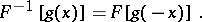Under the action of the Fourier transform linear operators on the original space, which are invariant with respect to a shift, become (under certain conditions) multiplication operators in the image space. In particular, the convolution of two functionsandgoes over into the product of the functions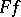and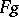: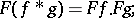and differentiation induces multiplication by the independent variable: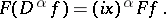In the spaces,, the operatoris defined by the formula (1) on the set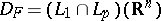and is a bounded operator from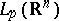into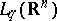,:

\begin{equation} \left\{\frac{1}{(2 \pi)^{n / 2}} \int_{\mathbf{R}^{n}}|(F f)(x)|^{q} d x\right\}^{1 / q} \leq\left\{\frac{1}{(2 \pi)^{n / 2}} \int_{\mathbf{R}^{n}}|f(x)|^{p} d x\right\}^{1 / p} \end{equation}

(the Hausdorff–Young inequality).admits a continuous extension onto the whole spacewhich (for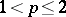) is given by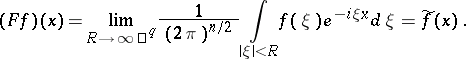(3)

Convergence is understood to be in the norm of. If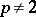, the image ofunder the action ofdoes not coincide with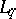, that is, the imbedding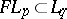is strict when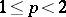(for the case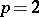see Plancherel theorem). The inverse operatoris defined on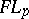by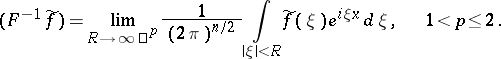The problem of extending the Fourier transform to a larger class of functions arises constantly in analysis and its applications. See, for example, Fourier transform of a generalized function.